# The Alon-Tarsi basis conjecture

 Importance: Medium ✭✭
 Author(s): Alon, Noga Linial, Nathan Meshulam, Roy
 Subject: Combinatorics » Matrices
Conjecture   If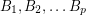are invertiblematrices with entries infor a prime, then there is asubmatrix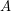of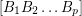so thatis an AT-base.
Definition: Ifis anmatrix over a field of characteristic, then we say thatis an Alon-Tarsi basis (or AT-basis) if the permanent of thematrix obtained by stacking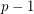copies ofis nonzero.
It follows from the Alon-Tarsi polynomial technique that ifis an AT-base then for every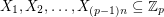of size 2 and for every, there exists a vector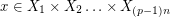so that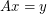(using the notation from A nowhere-zero point in a linear mapping,is (2,1)-choosable). It follows from this that every Alon-Tarsi base overis also an additive basis. Thus, the above conjecture, if true, would imply The additive basis conjecture. The following strengthening of this conjecture was suggested in [D]
Conjecture  (The strong Alon-Tarsi basis conjecture (DeVos))   Ifare invertiblematrices with entries in a field of characteristic, then we may partition the columns ofinto anmatrixand anmatrix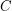so thatis an AT-base andis invertible.
In addition to implying the conjecture, above, if true, this conjecture would imply both The permanent conjecture and The choosability inconjecture.